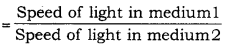# With respect to air, the refractive indices of water and benzene are 1.33 and 1.50 respectively

With respect to air, the refractive indices of water and benzene are 1.33 and 1.50 respectively. Calculate the refractive index of benzene with respect to water.

Refractive index of medium 1 with respect to mediumWe have, with respect to air, refractive index of water is 1.33 with respect to air, refractive index of benzene is 1.50

Therefore, with respect to water, refractive index of benzene